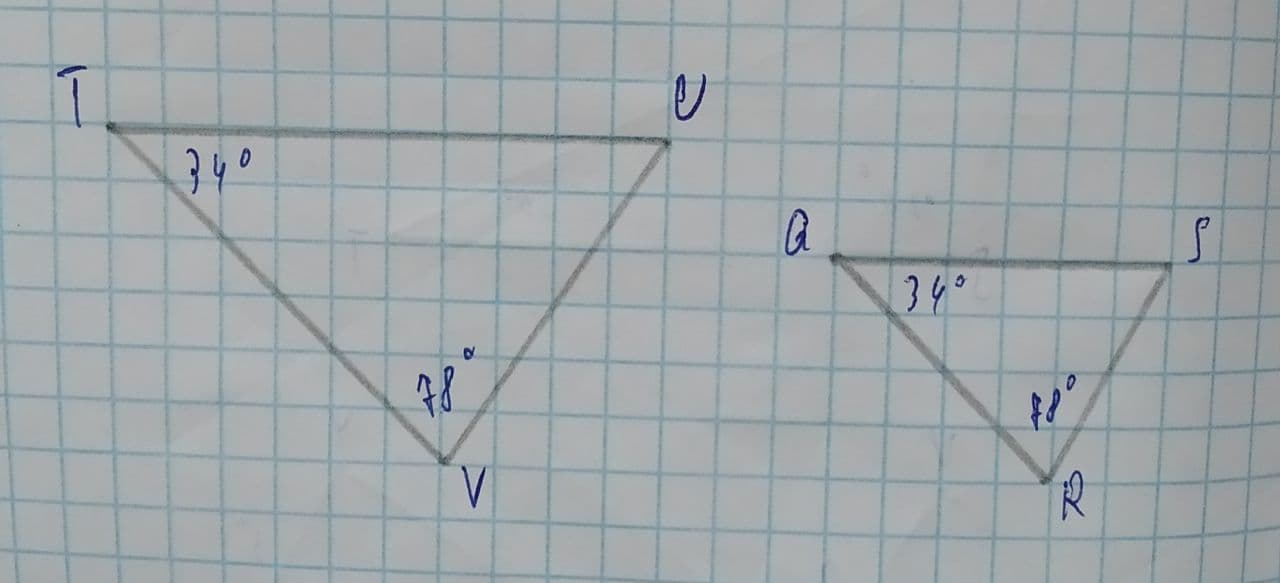Determine (if possible) the similarity postulate that proves the following two triangles are similar:fortdefruitI 2021-07-31 Answered
Determine (if possible) the similarity postulate that proves the following two triangles are similar:SAS similarity postulate
There is not enough information to determine whether the triangles are similar or not
AA similarity postulate
SSS similarity postulate

• Questions are typically answered in as fast as 30 minutes

Solve your problem for the price of one coffee

• Math expert for every subject
• Pay only if we can solve itl1koV
Step 1
In Triangle all angles total is 180 degre.
In given triangle both angles are equal then third angle is also equal so all angles are equal then triangle is similar. AA similarly
Step 2
AA similar